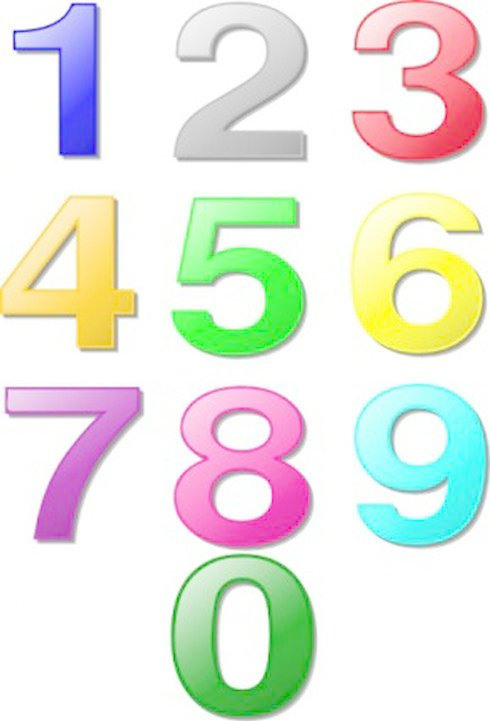# 数学超级”乘法口诀”是什么？有什么用？

小学刚开始学数学的时候，大家就开始背九九乘法表，因为这是数学学习的基础，所以我们学习数学的第一件事就是背诵乘法表，学生几乎都可以背过，并且能够熟练掌握，九九乘法表你知道了，但是超级”乘法口诀”你知道吗?这些口诀熟悉以后你的数学算术题会非常的容易，以下是秦学教育小编整理的，我们一起来看。一、十几乘十几：

口诀：头乘头，尾加尾，尾乘尾。

例：12×14=?

解: 1×1=1　　2+4=6　　2×4=8　12×14=168

注：个位相乘，不够两位数要用0占位。

二、头相同，尾互补(尾相加等于10)：

口诀：一个头加1后，头乘头，尾乘尾。

例：23×27=?

解：2+1=3　　2×3=6　　3×7=21　　23×27=621

注：个位相乘，不够两位数要用0占位。

三、第一个乘数互补，另一个乘数数字相同：

口诀：一个头加1后，头乘头，尾乘尾。

例：37×44=?

解：3+1=4　　4×4=16　　7×4=28　　37×44=1628

注：个位相乘，不够两位数要用0占位。

四、几十一乘几十一：

口诀：头乘头，头加头，尾乘尾。

例：21×41=?

解：2×4=8　　2+4=6　　1×1=1　　21×41=861

五、11乘任意数：

口诀：首尾不动下落，中间之和下拉。

例：11×23125=?

解：2+3=5　　3+1=4　　1+2=3　　2+5=7

2和5分别在首尾

11×23125=254375

注：和满十要进一。

六、十几乘任意数：

口诀：第二乘数首位不动向下落，第一因数的个位乘以第二因数后面每一个数字，加下一位数，再向下落。

例：13×326=?

解：13个位是3

3×3+2=11　　3×2+6=12　　3×6=18　　13×326=4238

注：和满十要进一。

是不是看完以后感觉其实算数也可以很简单，背过这些口诀以后，孩子在做数学题的时候，效率也会提高不少，所以口诀是非常有用的，一定要背下来。